### A.37 Maxwell’s wave equa­tions

This note de­rives the wave equa­tions sat­is­fied by elec­tro­mag­netic fields. The de­riva­tion will use stan­dard for­mu­lae of vec­tor analy­sis, as found in, for ex­am­ple, [41, 20.35-45].

The start­ing point is Maxwell’s equa­tions for the elec­tro­mag­netic field in vac­uum: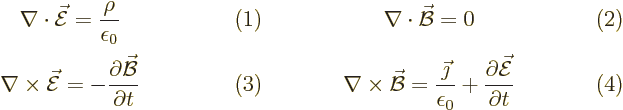Here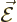is the elec­tric field,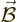the mag­netic field,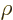the charge den­sity,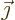the cur­rent den­sity,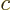the con­stant speed of light, and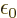is a con­stant called the per­mit­tiv­ity of space. The charge and cur­rent den­si­ties are re­lated by the con­ti­nu­ity equa­tion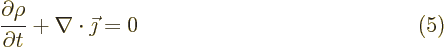To get a wave equa­tion for the elec­tric field, take the curl,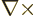, of (3) and ap­ply the stan­dard vec­tor iden­tity (D.1), (1) and (4) to get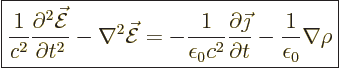(A.235)

Sim­i­larly, for the mag­netic field take the curl of (4) and use (2) and (3) to get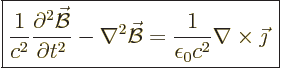(A.236)

These are un­cou­pled in­ho­mo­ge­neous wave equa­tions for the com­po­nents ofand, for given charge and cur­rent den­si­ties. Ac­cord­ing to the the­ory of par­tial dif­fer­en­tial equa­tions, these equa­tions im­ply that ef­fects prop­a­gate no faster than the speed of light. You can also see the same thing pretty clearly from the fact that the ho­mo­ge­neous wave equa­tion has so­lu­tions like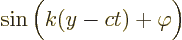which are waves that travel with speedin the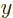-​di­rec­tion.

The wave equa­tions for the po­ten­tials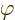and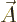are next. First note from (2) that the di­ver­gence ofis zero. Then vec­tor cal­cu­lus says that it can be writ­ten as the curl of some vec­tor. Call that vec­tor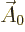.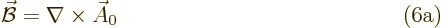Next de­fine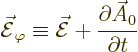Plug this into (3) to show that the curl of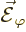is zero. Then vec­tor cal­cu­lus says that it can be writ­ten as mi­nus the gra­di­ent of a scalar. Call this scalar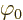. Plug that into the ex­pres­sion above to get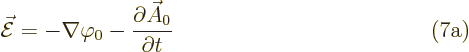Next, note that if you de­fine mod­i­fied ver­sionsandofandby set­ting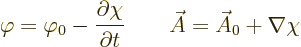where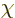is any ar­bi­trary func­tion of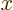,,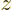, and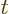, then still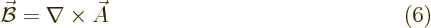since the curl of a gra­di­ent is al­ways zero, and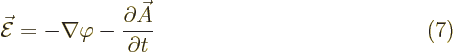be­cause the twoterms drop out against each other.

The fact that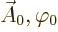and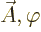pro­duce the same phys­i­cal fields is the fa­mous gauge prop­erty of the elec­tro­mag­netic field.

Now you can se­lectso that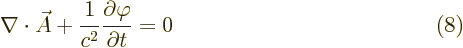That is known as the “Lorenz con­di­tion.” A cor­re­spond­ing gauge func­tion is a “Lorenz gauge.”

To find the gauge func­tionthat pro­duces this con­di­tion, plug the de­f­i­n­i­tions forandin terms ofandinto the left hand side of the Lorentz con­di­tion. That pro­duces, af­ter a change of sign,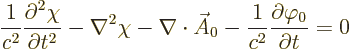That is a sec­ond or­der in­ho­mo­ge­neous wave equa­tion for.

Now plug the ex­pres­sions (6) and (7) forandin terms ofandinto the Maxwell’s equa­tions. Equa­tions (2) and (3) are sat­is­fied au­to­mat­i­cally. From (2), af­ter us­ing (8),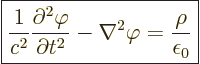(A.237)

From (4), af­ter us­ing (8),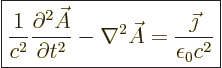(A.238)

You can still se­lect the two ini­tial con­di­tions for. The smart thing to do is se­lect them so thatand its time de­riv­a­tive are zero at time zero. In that case, if there is no charge den­sity,will stay zero for all time. That is be­cause its wave equa­tion is then ho­mo­ge­neous. The Lorenz con­di­tion will then en­sure that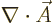is zero too.

In­stead of the Lorenz con­di­tion, you could se­lectto makezero. That is called the “Coulomb gauge” or “trans­verse gauge” or “trans­verse gauge.” It re­quires thatsat­is­fies the Pois­son equa­tion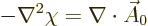Then the gov­ern­ing equa­tions be­come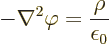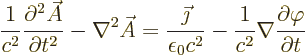Note thatnow sat­is­fies a purely spa­tial Pois­son equa­tion.